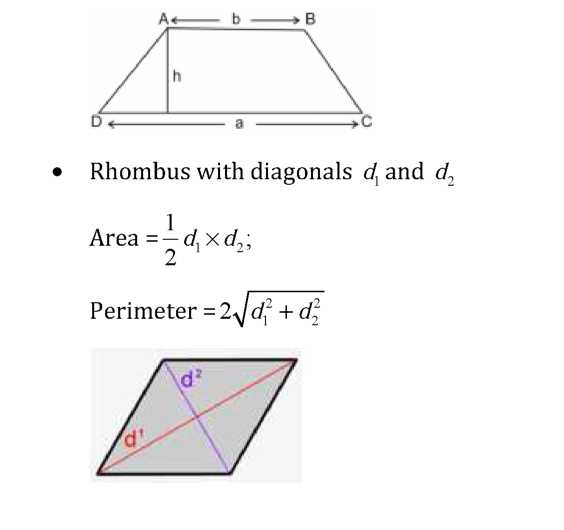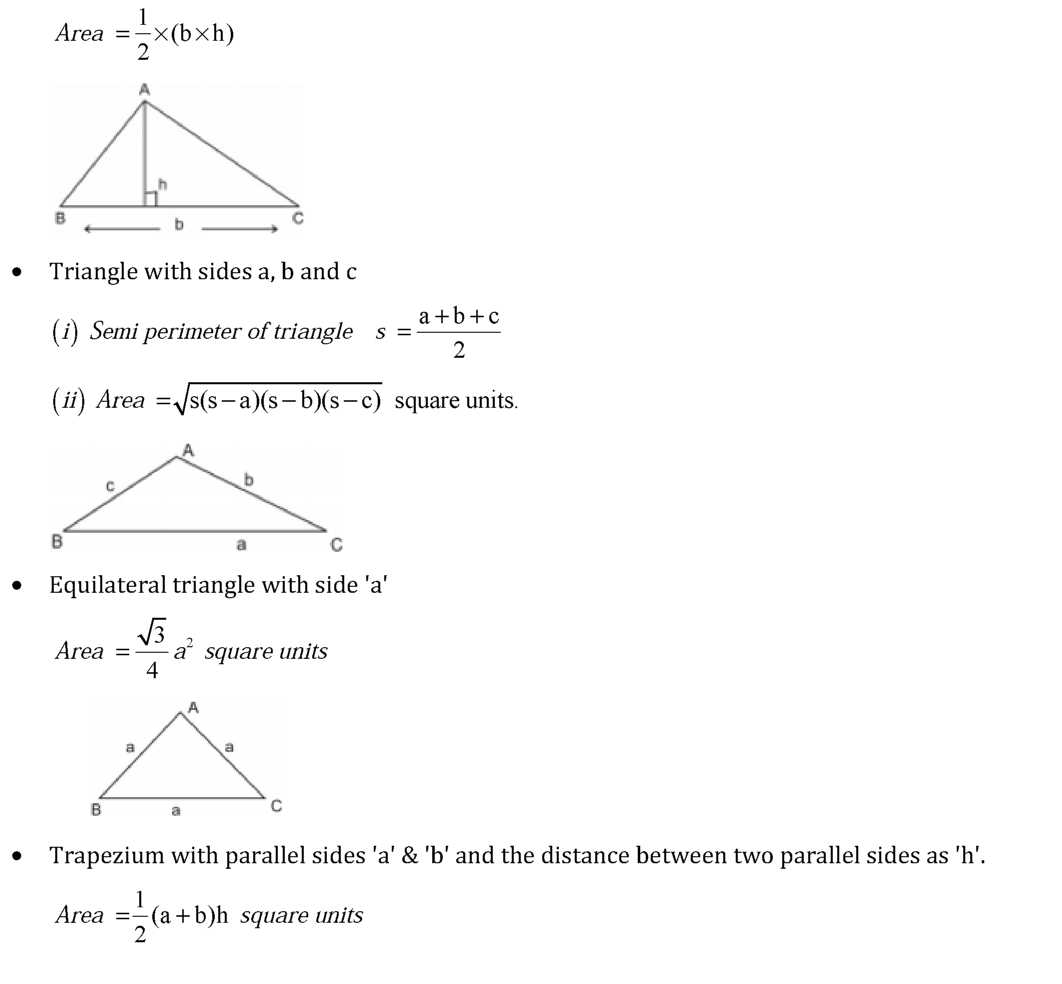# Chapter 12 Heron’s Formula Notes for Class 9th Maths

0
1874Chapter 12 Heron’s Formula

1. Area of a Triangle – by Heron’s Formula
2. Application of Heron’s Formula in finding Areas of Quadrilaterals
• Triangle with base ‘b’ and altitude ‘h’ isPrevious articleChapter 11 Constructions Notes for Class 9th Maths